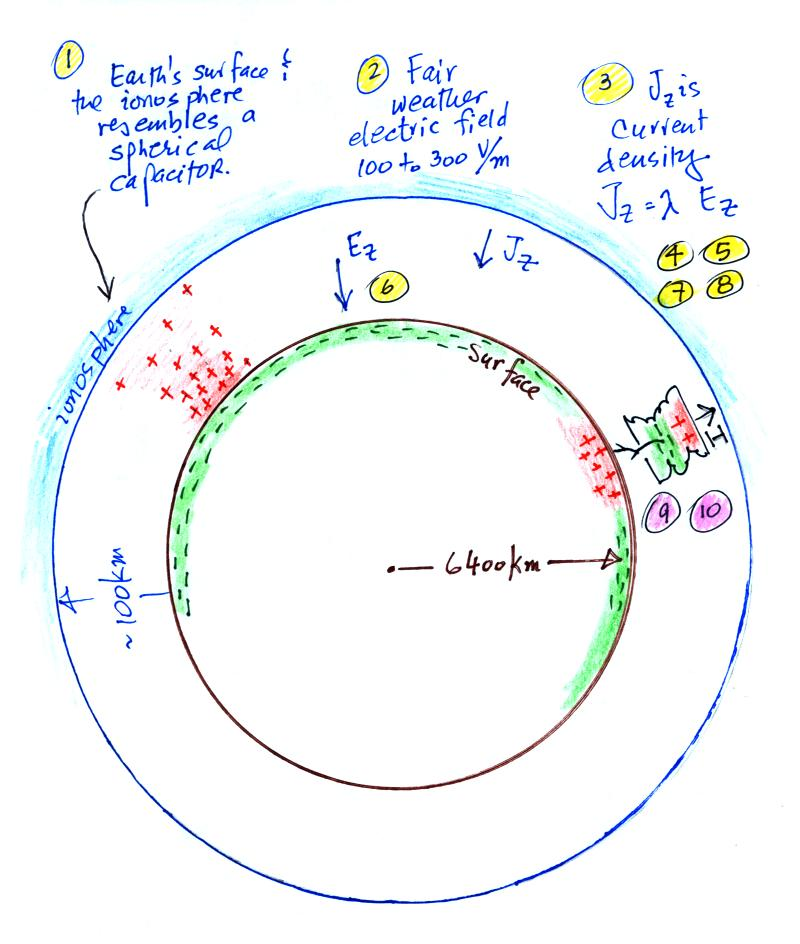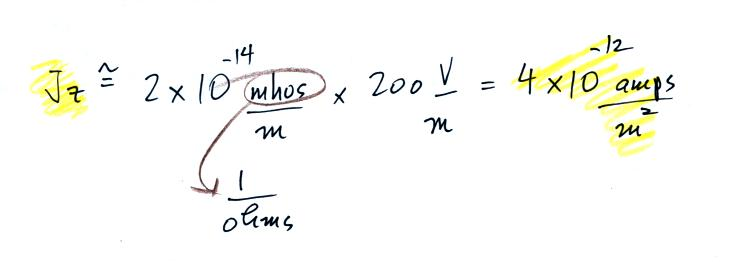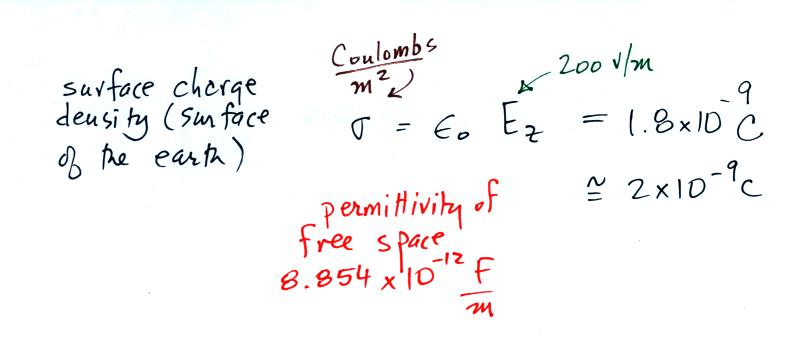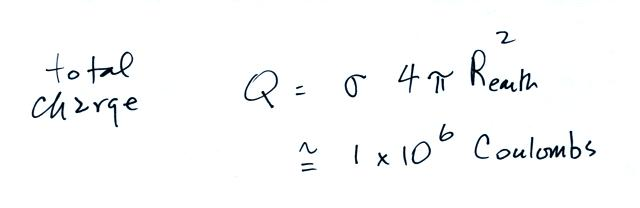We'll begin this course with a quick look at the global atmospheric electrical circuit.  This is a way to introduce some of the topics that we will be covering in more depth during the remainder of this class.

The figure below shows the earth's surface and the bottom 100 km or so of the earth's atmosphere - the part extending from the ground up to the bottom of the ionosphere.  In the ionosphere there is enough ionization of air (mainly by ultraviolet radiation) to make the atmosphere an electrically conducting layer.  The figure is not drawn to scale, the 100 km thick layer of atmosphere has been greatly magnified.Point 1  Together, the earth's surface and the ionosophere resemble a charged spherical capacitor, i.e. two oppositely charged conducting electrodes with an insulator in between.   The ground is normally negatively charged during fair weather.  Positive charge is found in the air between the ground and the ionosphere (the charge would normally be found on the second electrode in a typical capacitor).  The positive charge is attached to small particles in the air (aerosols) and is relatively immobile (compared to air molecules due to the large size and large inertia of the particles).  These are called "large ions."  Most of the positive charge is found near the ground.

Point 2  Negative charge on the ground and positive charge in the air above means that there is a downward pointing, 100 to 300 volts/meter (V/m) electric field (E field) at the ground during normal fair weather conditions.  Soil and ocean water are much better conductors than air so we'll often assume that the ground is a perfect conductor in many of the problems that we look at.  In that case the E field will be perpendicular to the ground.
Also because the atmosphere is much thinner than the radius of the earth, we will usually be able to just consider the ground to be flat and ignore the fact that it is curved.  That's why we used Ez (cartesian coordinate system) in the picture instead of Er (spherical coordinates).

Note that the ground is normally positively charged underneath a thunderstorm (Point 9).  The electric field at the ground under a thunderstorm are normally more intense, 1000s of V/m.

Points 3 & 4  Air is not a perfect insulator, it does have a very small but finite conductivity.  A very weak current flows from the ionosphere to the ground.
Conduction of electricity in the atmosphere is a little different from what happens in a wire.  In a wire it is the motions of free electrons alone that carry current from one point to another.  In the atmosphere charge carries of both polarites carry current.  These charge carriers are called "small ions" and consist of charged clusters of molecules that are much smaller and more mobile than large ions.  During the class we will have a look at how small ions are created (and destroyed).Current flowing to the ground is denoted
Jz in the figure.  J stands for current density which has units of amperes/meter2A conduction current term which depends on the strength of the electric field (E) and the conductivity of the air (lambda in the figure below) is often the main component of Jz .We'll find that Ez decreases, conductivity increases, and current density remains about constant with increasing altitude.

Point 5  We can assume reasonable values for the strength of the "fair weather" electric field and the conductivity of the air to estimate Jz.We can multiply this current density by the area of the earth's surface to determine to total current flowing between the ionosphere and the earth's surface.Point 6  Let's step backward briefly.  An electric field of 200 V/m would mean there would be a 400 volt difference between the ground and a point 2 meters above the ground.  That's about a 400 volt difference between our head and our toes when we step outside.  Why don't we feel this?Air has a very low conducitivity (high resistance), a very weak current flowing through air can produce a large potential difference.  The resistance of a human body is much lower (I don't really know what the resistance of a human body is, perhaps 1000 ohms up to as much as 100,000 ohms depending on how wet or dry the  body is).  Compared to air the person is effectively a short circuit and there really is very little or no head-to-toe potential difference.

Point 7  The potential of the ionosphere ranges from 150 kV to 600 kV relative to the earth's surface (see Table 15.1 in The Earth's Electrical Environment )  We'll use an average value of 280,000 volts.

We can divide the surface-ionosphere potential difference by the current flowing between the ionosphere and the surface to determine an effective resistance of the atmosphere.Point 8  The following equation shows the relationship between surface charge density (Coulombs per unit area) and electric field at the surface of the earth (we'll derive this expression soon in this class, it's a simple application of Gauss' Law)We'll multiply by the area of the surface of the earth to determine the total charge on the earth's surfaceThe earth's surface is charged, but a weak current flows through the atmosphere to the earth trying to neutralize the charge on the earth. The following calculation shows that it wouldn't take very long for the current flowing between the ionosphere and the ground, I, to neutralize the charge on the earth's surface, Q.It would only take about 10 minutes to discharge the earth's surface.  This doesn't happen however.  The obvious question is what maintains the surface-ionsphere potential difference?  What keeps the earth-ionosphere spherical capacitor charged up?Point 9  The original answer was lightning.  Most cloud-to-ground lightning carries negative charge to the ground.

At some point it became clear that lightning alone wasn't enough.  The thinking then became thunderstorms in general.  Point (b) shows an upward current flowing from the top of the thunderstorm and also from point discharge currents on the ground.  But these currents aren't quite sufficient either.

The current thinking is that thunderstorms and non-thundery (Point (c) above) but electrified clouds are needed to produce sufficient charging current.

Points 1-8 in the figure at the beginning of today's notes constitute what might be called "fair weather atmospheric electricity."  We'll spend a significant portion of this class discussing this topic.

Point 10  Most of the remainder of the class will be devoted to stormy weather electricity, i.e. thunderstorms, lightning, and related topics.  We'll look at how thunderstorms become electrified (doesn't it seem surprising that electrical charge is created and separated in the cold wet windy interiors of thunderstorms?).  We'll spend quite a bit of time looking at the sequence of events that make up negative cloud-to-ground lightning.  We'll also look at other types of lightning (intracloud lightning, positive cloud-to-ground lightning, upward and triggered lightning).  We'll look at how lightning current characteristics can be measured.  This is important because some knowledge of lightning currents characteristics is needed to to design effective lightning protection equipment.  Lightning protection of structures and electrical systems is something else we'll cover.  We'll also look at new ground- and satellite-based sensors being used to detect lightning as it occurs around the globe.

We try to include as many basic demonstrations and examples of working instrumentation used in thunderstorm and lightning research in the classroom version of this course because they are entertaining and educational.  As much as possible we'll try to do the same in this online course.

Along those lines, the flow of electricity between the ionosphere and the surface of the earth in some respects resembles the visible discharges in a plasma globe.  Some photos are shown below (source).You'll find a clear and basic explanation of how plasma globes work here.